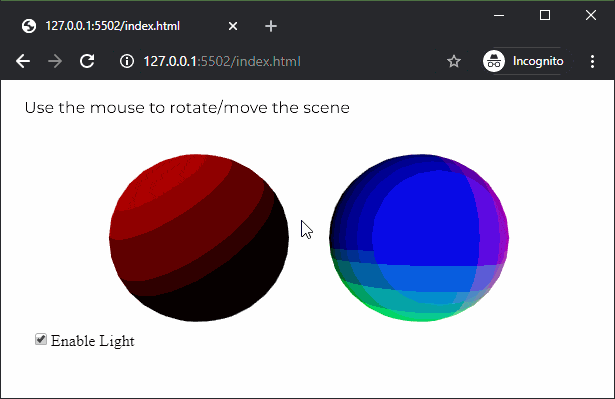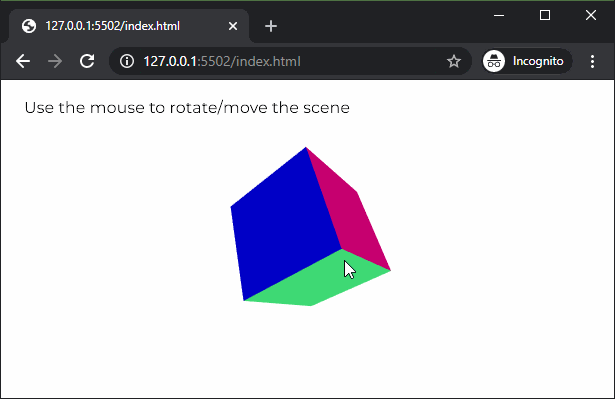# p5.js | normalMaterial() Function

• Last Updated : 27 Apr, 2020

The normalMaterial() function in p5.js is used to create a normal material for an object. A normal material is not affected by any light and also does not reflect any light. The surface facing the x-axis becomes red, the surface facing the y-axis becomes green and the surface facing the z-axis becomes blue. It is usually used as a placeholder material for debugging.

Syntax:

`normalMaterial()`

Parameters: This function does not accept any parameters.

Below example illustrates the normalMaterial() function in p5.js:

Example 1: This example shows that the normal material is not affected by light.

 `let newFont; ` `let hasLight = ``true``; ` ` `  `function` `preload() { ` `  ``newFont = loadFont(``'fonts/Montserrat.otf'``); ` `} ` ` `  `function` `setup() { ` `  ``createCanvas(600, 300, WEBGL); ` `  ``textFont(newFont, 16); ` ` `  `  ``lightCheckbox = createCheckbox(``'Enable Light'``, ``true``); ` `  ``lightCheckbox.position(30, 250); ` `  ``lightCheckbox.changed(() => hasLight = !hasLight); ` `} ` ` `  `function` `draw() { ` `  ``background(``'white'``); ` `  ``fill(``'black'``); ` ` `  `  ``text(``"Use the mouse to rotate/move the scene"``, -285, -125); ` `  ``noStroke(); ` `  ``orbitControl(); ` ` `  `  ``// Enable lights when the checkbox is checked ` `  ``if` `(hasLight) ` `    ``directionalLight(color(``'red'``), height / 2, width / 2, -250); ` ` `  `  ``// Draw the sphere which uses ambient material ` `  ``ambientMaterial(255); ` `  ``translate(-100, 0, 0); ` `  ``sphere(80); ` `  ``translate(100, 0, 0); ` ` `  `  ``// Draw sphere which uses normal material ` `  ``normalMaterial(); ` `  ``translate(100, 0, 0); ` `  ``sphere(80); ` `  ``translate(-100, 0, 0); ` `} `

Output:Example 2: This example shows the colors for the different axes of the normal material.

 `let newFont; ` `let hasNormalMaterial = ``true``; ` ` `  `function` `preload() { ` `  ``newFont = loadFont(``'fonts/Montserrat.otf'``); ` `} ` ` `  `function` `setup() { ` `  ``createCanvas(600, 300, WEBGL); ` `  ``textFont(newFont, 16); ` `} ` ` `  `function` `draw() { ` `  ``background(``'white'``); ` `  ``fill(``'black'``); ` ` `  `  ``text(``"Use the mouse to rotate/move the scene"``, -285, -125); ` `  ``// text("Select directional light color", -285, -100); ` `  ``shininess(10); ` `  ``noStroke(); ` `  ``orbitControl(); ` ` `  `  ``// Use the normal material ` `  ``normalMaterial(); ` ` `  `  ``// Create the box ` `  ``rotateX(60) ` `  ``rotateY(120) ` `  ``rotateZ(60) ` `  ``box(100); ` `} `

Output:Online editor: https://editor.p5js.org/

My Personal Notes arrow_drop_up
Recommended Articles
Page :